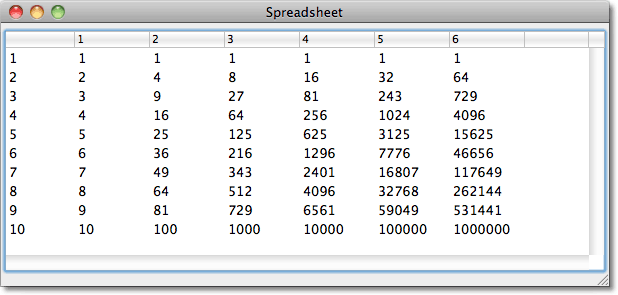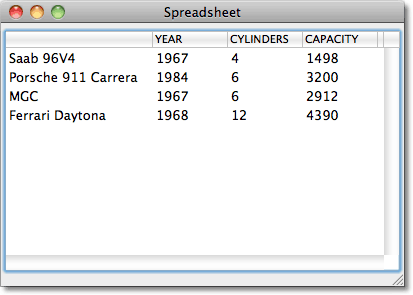This example shows how to use the CAPI multi-column-list-panel to create a general purpose multi-column table for displaying data. The general call is:

`(spreadsheet rows columns cell-fn)`

where rows is a list of the items to be displayed down the vertical axis, columns is a list of items to be displayed across the top of the table, and cell-fn is a function of two arguments to calculate the contents of each cell.

Complete listing

### Examples

This simple example calculates the powers of the numbers 1 to 10:

`(spreadsheet '(1 2 3 4 5 6 7 8 9 10) '(1 2 3 4 5 6) #'expt)`

This displays:You can click on the heading of each column to sort the table in ascending or descending order by that column. The spreadsheet correctly sorts each column based on whether its content is a string or number.

The command can also take a row-label-fn and a column-label-fn, which are used to calculate the labels along the left and top of the table. For example, suppose we had a class defined as follows:

```(defclass classic-car ()
((name :initarg :name :accessor name)
(year :initarg :year)
(cylinders :initarg :cylinders)
(capacity :initarg :capacity)))
```

We could define some instances as follows:

```(defparameter *cars*
(list
(make-instance 'classic-car :name "Saab 96V4" :year 1967 :cylinders 4 :capacity 1498)
(make-instance 'classic-car :name "Porsche 911 Carrera" :year 1984 :cylinders 6 :capacity 3200)
(make-instance 'classic-car :name "MGC" :year 1967 :cylinders 6 :capacity 2912)
(make-instance 'classic-car :name "Ferrari Daytona" :year 1968 :cylinders 12 :capacity 4390)))```

Then to display a table of all the objects and their slots we simply call:

`(spreadsheet *cars* '(year cylinders capacity) #'slot-value   :row-label-fn #'name :column-label-fn #'string-capitalize)`

This displays:### The definition

Here's the full definition:

```(defun spreadsheet (rows columns cell-fn
(column-label-fn #'identity))
"Draws a table by applying cell-fn to each combination of an element from the
rows and columns lists."
(let* ((sequence (map
'list
#'(lambda (row)
(cons (funcall row-label-fn row)
(map
'list
#'(lambda (column) (funcall cell-fn row column)) columns))) rows))
(table (make-instance 'capi:multi-column-list-panel
:items sequence
:columns
(cons '(:width 144 :title :| |)
(map 'list
#'(lambda (column)
(list
:width 72
:title (intern
(princ-to-string
(funcall column-label-fn column))
:keyword)))
columns))
:callback-type :collection-data
columns
:column-label-fn column-label-fn)
:external-min-width (+ 144 (* 76 (length columns)))
:external-max-width nil :external-min-height 240))
(window (make-instance 'capi:interface
:title title
:layout
(make-instance 'capi:column-layout :description (list table)))))
(capi:display window)))```

This creates a multi-column-list-panel with the following parameters:

:items is set to sequence, which is a list of the rows of the table. Each row is a list consisting of the row label, followed by the items in that row.

:columns is set to a list specifying the width and title of each column. Each column is identified by a keyword, which is both used as the title of the column, and to label the sorting descriptions for the columns. The first column has the label :| |, a keyword with a space as its name.

:sort-descriptions uses spreadsheet-sort-descriptions to generate the sorting descriptions for each column. It returns a list of sorting-descriptions, one for each column, with :type set to the keyword labelling the column:

```(defun spreadsheet-sort-descriptions (columns &key (column-label-fn #'identity))
(let ((n 0))
(cons
(capi:make-sorting-description
:type :| |
:sort (test-nth 0)
:reverse-sort (test-nth 0 t))
(map 'list #'(lambda (column)
(incf n)
(capi:make-sorting-description
:type (intern (princ-to-string (funcall column-label-fn column)) :keyword)
:sort (test-nth n)
:reverse-sort (test-nth n t)))
columns))))```

The sorting-description sorts the whole row based on the nth item. It uses the routine test-nth which returns a function to compare the nth items of the two lists passed to it as the two parameters x and y:

where test-nth is defined as follows:

```(defun test-nth (n &optional reverse)
#'(lambda (x y)
(funcall (if (numberp (funcall #'nth n x)) #'> #'string-greaterp)
(funcall #'nth n (if reverse y x))
(funcall #'nth n (if reverse x y)))))```

This routine test-nth looks at the items being compared and uses > or string-greaterp as appropriate to sort the items, so it works correctly even if the columns contain different types of data.